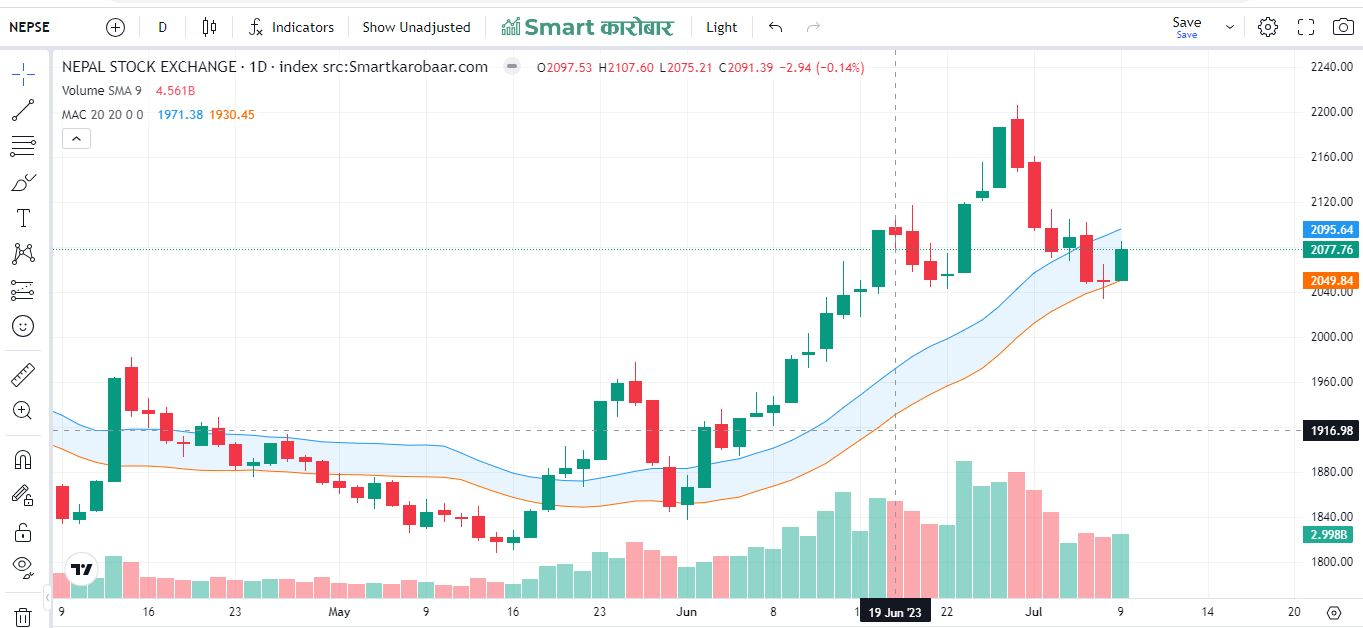# Moving Average Channel (MAC)

The moving average channel (MAC) is a method that was developed and refined over twenty years ago. It is very useful and effective in stocks and futures. It offers a specific method for determining the initial stop-loss for a trade once there has been a setup and a trigger.

It defines the exact procedure for different position sizes and commands on how and when to trail stop-losses.

By applying an Channel to the moving average, some of these  trades can be avoided, and traders can increase their profits.MAC trading has been a favorite tool among technical analysts for years, and incorporating that technique with MAs makes for a useful combination.

How does moving average channel calculated ?

A simple moving average is calculated by adding the closing prices of a stock over a specified number of time periods, usually days or weeks. As an example, a 10-day simple moving average is calculated by adding the closing prices over the last 10 days and dividing the total by 10.

Average = MA(PRICE, n) Upper Channel = Average + m * SD[Average, x] Lower Channel = Average - p * SD[Average, x] where n = MA Period x = Std Dev Period m = number of Standard Deviations (upper) p = number of Standard Deviations (lower) SD = Standard Deviation over last x periods